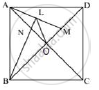Share

In a Square Abcd, Its Diagonals Ac and Bd Intersect Each Other at Point O. Show That: (I) ∠Onl + ∠Oml = 180 (Ii)∠Bam = ∠Bma (Iii) Alob is a Cyclic Quadrilateral - ICSE Class 10 - Mathematics

Question

In a square ABCD, its diagonals AC and BD intersect each other at point O. the bisector of angle DAO meets BD at point M and the bisector of angle ABD meets AC at N and AM at L. Show that:
(i) ∠ONL + ∠OML = 180
(ii)∠BAM = ∠BMA
(iii) ALOB is a cyclic quadrilateral

Solution

ABCD is a square whose diagonals AC and BD intersect each other at right angles at O.i)

∴ ∠AOB = ∠AOD = 90°

In Δ ANB ,

∠ANB = 180° - (∠NAB + ∠NBA)

⇒ ∠ANB = 180° - (45° + 45°/2)(NB is bisector of ∠ABD)

⇒ ∠ANB = 180° - 45° - (45°)/2 = 135° - (45°)/2

But, ∠LNO = ∠ANB (vertically opposite angles)

∴ ∠LNO = 135° - (45°)/2      ..................(i)

Now in  ΔAMO,
∠AMO = 180°  - ( ∠AOM + ∠OAM)

⇒ ∠AMO = 180° - (90 + (45°)/2) (MA is bisector of ∠DAO)

⇒ ∠ AMO = 180° - 90° - (45°)/2 = 90° - (45°)/2   ...................(ii)

Adding (i) and (ii)
∠LNO  + ∠AMO  = 135 - (45°)/2 + 90° - (45°)/2
⇒ ∠LNO + ∠AMO  = 225° -  45°  = 180°
⇒  ∠ONL +  ∠OML =  180°

ii)
∠BAM = ∠BAO + ∠OAM
⇒ ∠BAM  = 45° +  (45°)/2 = 67  (1°)/2
And
⇒ ∠BMA = 180° - (∠AOM + ∠OAM)
⇒ ∠BMA = 180° - 90° - (45°)/2 =  90° - (45°)/2 =  67(1°)/2
∴ ∠BAM =  ∠BMA
iii) In quadrilateral ALOB,
∵ ∠ABO + ∠ALO = 45°  + 90° + 45°  = 180°
Therefore, ALOB is a cyclic quadrilateral.

Is there an error in this question or solution?

APPEARS IN

Solution In a Square Abcd, Its Diagonals Ac and Bd Intersect Each Other at Point O. Show That: (I) ∠Onl + ∠Oml = 180 (Ii)∠Bam = ∠Bma (Iii) Alob is a Cyclic Quadrilateral Concept: Cyclic Properties.
S